Learn US

# Absolute Value Equations

86 views

 1 Introduction 2 Absolute Value Equations 3 Absolute Value Equations Examples 4 How to solve absolute value equations 5 Solving absolute value equations 6 Summary 7 FAQs 8 External References

23 December 2020

## Introduction

If you're trying to solve an equation containing a variable that's inside absolute value bars, you'll need to slightly change your technique. Here's why we want to do so: Such equations may have two answers, instead of just one! That might be initially exciting (like finding out that while you had one secret admirer, you actually have two), once you figure it all out in the end, everything makes sense.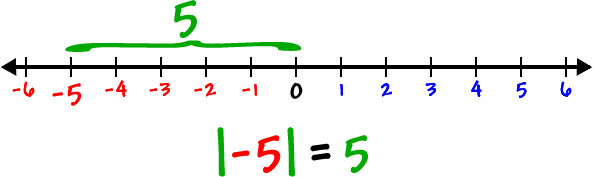### How to solve equations with absolute value?

Here's what to do if you found  an equation whose poor, defenseless variable is trapped in absolute value bars and you are working towards solving absolute value equations:

### 1. Isolate the absolute value expression

Just like you isolated the variables before, this time isolates that entire expression that falls between the absolute value bars. Follow the same steps,  you can start by adding or subtracting values out of the way and finish by eliminating a coefficient if the expression has one coefficient.

### 2. Create two new equations.

Here's the tricky part. You're actually going to make two completely separate equations from the original equation. The first equation must look like the original, just without the bars on it. The second should look like the first, only take the opposite of the right side of the equation. This might sound tricky, but trust me, it's easy.

3. Now solve the new equations to get your answer(s). Both of the solutions you get are answers to the original absolute value equation.

You had created two separate equations because absolute values change two different values (any number and its opposite) into the same values. Sometimes to remind me that absolute value equations require two separate parts, I sometimes imagine that those absolute value bars are little bars of dynamite that blow that original equation in half, creating two different pieces.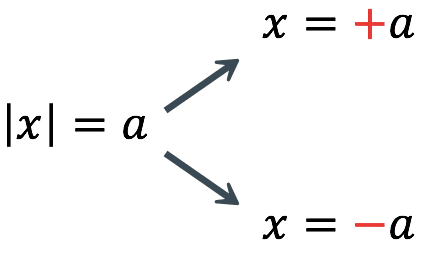Now that you get the idea, let me show you how to handle the explosives correctly.

If you ever want to read it again as many times as you want, here is a downloadable PDF to explore more.

## Absolute Value Equations

An absolute value equation is an equation that contains an absolute value expression. The equation. |x|=a. Has two solutions x = a and x = -a because both numbers are at the distance a from 0. Now, we will learn how to solve an absolute value equation. To solve an equation such as \begin{align}8 = \left| {2x - 6} \right|,\end{align} we notice that the absolute value will be equal to 8 if the quantity inside the absolute value is 8 or -8. This generates two different equations we can solve independently.

\begin{align}2x - 6 &= 8\\2x &= 14\\x &= 7\\\end{align}    or     \begin{align}2x - 6 &= - 8\\2x &= - 2\\x &= - 1\end{align}

Knowing how to solve problems with absolute value functions is recommended. For example, we may need to look at numbers or points on a line that is at a specified distance from a given point.
An absolute value equation is an equation in which an unknown variable appears in absolute value bars. For example,

\begin{align}\left| x \right| &= 4,\\\left| {2x - 1} \right| &= 3,\text{or}\,\\\left| {5x + 2} \right| - 4 &= 9\end{align}

## Absolute value equations examples

 Example 1

Determine all values,  x, within a distance of 4 from number 5.

### Solution

We need to solve the distance between x  and 5 to be less than or equal to 4. The distance can be represented using the absolute value, giving the expression

|x−5|≤4

 Example 2

A 2010 poll reported 75% of Americans to believe that people who are gay should be able to serve in the US military, with a reported margin of error of 3%. The margin of error tells us how far off the actual value could be from the survey value (Technically, the margin of error usually means that the surveyors are 95% confident that actual value falls within this range.). Determine the set of possible values using absolute values.

### Solution

Since we want  to know  of the difference between the actual percentage,  p , and the given percentage to be less than 3%,

|p−75 |≤ 3

## How to solve absolute value equations

For real numbers, A and B, an equation of the form \begin{align}\left| A \right| = B,\end{align}  with \begin{align}B \ge 0,\end{align} will have solutions when  A = B or A  =-B If  B < 0 the equation\begin{align}\left| A \right| = B\end{align} has no solution. With the help of  formula for an absolute value function, find the horizontal intercepts of its graph. Certain steps are to be followed when solving absolute value equations:

• Isolate the absolute value term.
• Use \begin{align}\left| A \right| = B\end{align} to write\begin{align}A = B\end{align}  or \begin{align}-A = B\end{align}assuming B > 0
• Solve for x
 Example 1

For the function \begin{align}f(x) = \left| {4x + 1} \right| - 7,\end{align} find the values of x such that \begin{align}f(x) = 0.\end{align}

\begin{align}&0 = \left| {4x + 1} \right| - 7\qquad\qquad\qquad\qquad\,\,\rm{substitue}\,0\,\rm{for}\,f(x) = 0.\\&7 = \left| {4x + 1}\right|\qquad\qquad\qquad\qquad\qquad\text{Isolate the absolute value on one side of the equation.}\end{align}

\begin{align}&7 = 4x + 1\\&6 = 4x\\&x = \frac{6}{4} = 1.5\end{align}       Or         \begin{align}& - 7 = 4x + 1\,\qquad\qquad\rm{Break}\,\,{\mathop{\rm into two separate equations and above}\nolimits} \\& - 8 = 4x\\&x = \frac{{ - 8}}{4} = - 2.\end{align}

The function outputs 0 when \begin{align}x = \frac{3}{2}\end{align}  or  \begin{align}x = - 2.\end{align}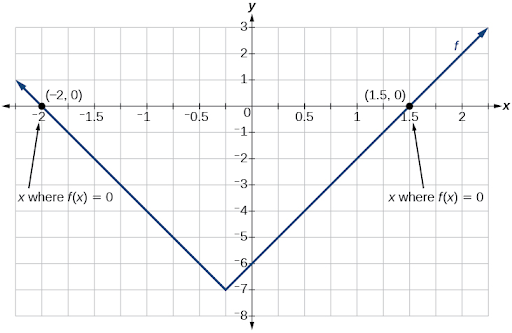For the function \begin{align}f(x) = \left| {2x - 1} \right| - 3,\end{align} find the values of x such that \begin{align}f(x) = 0.\end{align}
x = -1 or x = 2
Do we always expect two answers while solving \begin{align}\left| A \right| = B?\end{align}
No. We may get one, two,  sometimes no answers. For  this example, there is no solution for this equation \begin{align}2 + \left| {3x - 5} \right| = 1.\end{align}

 Example 2

Solving the absolute value equations for the function graphed.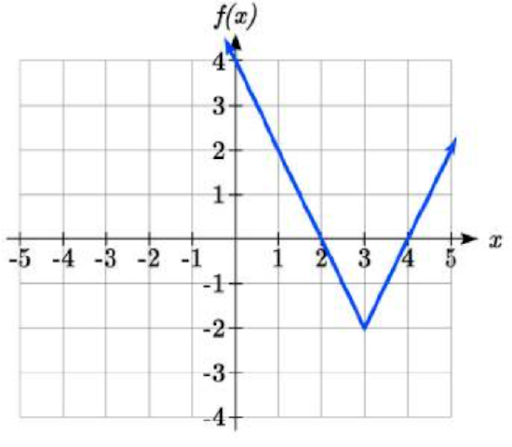### Solution

The absolute value function changes direction at the origin, so this graph has been shifted to the right 3 and down 2 from the basic function.

We might also notice that the graph appears little stretched since the linear portions have slopes of 2 and -2. From this information we could write  the equation in two ways:

f(x) = 2|x−3| − 2,  making  the stretch as a vertical stretch

f(x) = |2(x−3)| − 2, making  the stretch as a horizontal compression

These equations are algebraically equivalent – the stretch for an absolute value function can be written as a vertical or horizontal stretch/compression.

If you had not been able to find the stretch based on the slopes of the lines, you can solve for the stretch factor by putting in a known pair of values for x and f(x)

f(x) = a|x−3| − 2

Now substituting in the point (1, 2)

2 = a|1−3| − 2|\
4 = 2a
a = 2

## Solving Absolute Value Equations

Now while solving absolute value equations, one must keep some steps in mind. When answering questions like how to solve an equation like  8=|2x−6|, the absolute value will be equal to eight if the quantity inside the absolute value were 8 or -8. This leads to two different equations we can solve independently:

2x − 6 = 8 or 2x − 6 = −8

2x = 14  2x = −2

x = 7 x = −1

An equation in  the form  of  |A| = B, with  B ≥ 0, must  have solutions when

A = B or A = −B

 Example 1

Solve  the horizontal intercepts of the graph of  f(x) = |4x+1| − 7

### Solution

The horizontal intercepts will occur when f(x) = 0. Solving the absolute value equations,

0 = |4x+1| − 7
Keep the absolute value on one side of the equation

7 = |4x+1|

Now we can split  this into two separate equations:

7 = 4x+1    or  −7 = 4x+1

6 = 4x or −8 = 4x

x = 6/4 = 3/2
x = −8/4 = −2

The graph has two horizontal intercepts, at  x = 3/2  and  x = −2

 Example 2

Solving absolute value equation  1 = 4|x−2| + 2

### Solution

Keeping the absolute value on one side the equation,

1 = 4|x−2|+2

−1 = 4|x−2|

−1/4 = |x−2|
Now this equation has no solutions – the absolute value always returns a positive value, so it is impossible for the absolute value to equal negative value.

 Example 3

Determine the horizontal & vertical intercepts for the function f(x) = −|x+2|+3

### Solution

Solving Absolute Value Inequalities

When absolute value inequalities are written to describe a set of values, for example, the inequality  |x−5|≤4, it is sometimes needed to express this set of values without the absolute value, either using inequalities or using interval notation.

We will explore two ways  to solving absolute value inequalities:

• Using the graph
• Using test values
 Example 4

Solving absolute value equations of this  |x−5|≤4

### Solution

With both ways, we will need to know first where the corresponding equality is true. In this case, we find where is |x−5|=4 located. We do this as the absolute value is a  friendly function with no breaks, so the only way the function values can switch from being less than 4 to being greater than 4 is by passing through where the values equal 4.
Solve  |x−5|=4,

x−5=4  x=9 or x−5=−4   x=1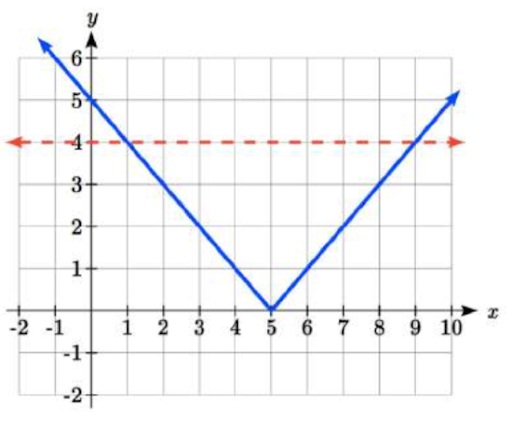To use a graph, we can make a sketch of the function f(x)=|x−5|  which will help us see where the outputs are 4, the line g(x)=4  could also be.On the graph, we can see that indeed the output values of the absolute value are equal to 4 at  x=1  and  x=9. Based on the shape of the graph, we can find the absolute value is less than or equal to 4 between these two points when  1≤x≤9. In interval notation, this can be the interval [1,9].

As an alternative to graphing, after determining that the absolute value is equal to 4 at  x=1  and  x=9, we know the graph only changes from being less than 4 to greater than 4 at these values. This divides the number line up into three intervals:  x<1,  1<x<9, and x>9. To
determine who sketched the function is less than 4, we could pick a value in each interval and see if the output is less than or greater than 4.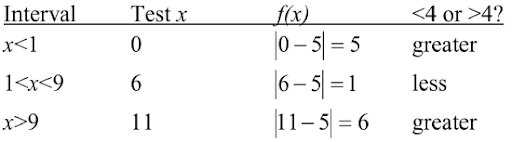Hence  1≤x≤9  is the only interval in which the output at the test value is less than 4, we can conclude the solution to  |x−5|≤4  is  1≤x≤9.

 Example 5

Given the function f(x)=−1/2|4x−5|+3, find out what x  values the function values are negative.

### Solution

We are trying to find where f(x)<0, which is when  −1/2|4x−5|+3<0. We begin by keeping isolating the absolute value:

−1/2|4x−5|<−3

when we multiply both sides by -2, it reverses the inequality

|4x−5|>6

Now we solve for the equality  |4x−5|=6
4x−5=6   4x=11
x=11/4 or 4x−5=−6
4x=−1    x=−1/4

We can now either choose test values or sketch a graph of the function to determine which intervals the original function value is negative. Notice that it is not very important exactly what the graph looks like, as long as we know that it crosses the horizontal axis at  x=−1/4  and  x=11/4 and that the graph has been reflected vertically.

From the graph of the function, we can see the function values are negative to the left of the first horizontal intercept at  x=−1/4 and negative to the right of the second intercept at  x=11/4. This gives us the solution to inequality:

x < −1/4 or  x > 11/4

In interval notation, this would be  (−∞,−1/4)⋃(11/4,∞)

 Example 6

Solving absolute value equations of  −2|k−4|≤−6

### Solution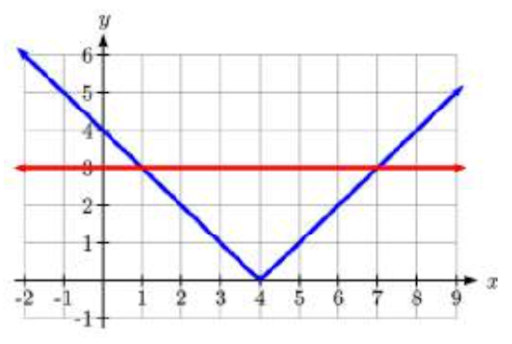−2|k−4|≤−6

|k−4| ≥ 3

Solving the equality  |k−4| = 3, k – 4 = 3 or k – 4 = –3, so k = 1 or k = 7.Using a graph or test values, we can  find  the intervals that satisfy the inequality are  k≤1  or  k≥7; in interval notation, this would be  (−∞,1]∪[7,∞)

## Summary

Students solving absolute value equations have a few options other than doing them like the examples above. It can be quite easy to use an absolute value equation graph to solve and check the answers. For students, using a solving absolute value equations calculator can add little advantage to check their answers as they learn how to do these problems.

Solving for absolute value with numbers, equations, and variables involves understanding the order in which the problem is solved and realizing how the absolute value works. Take the time to do solving absolute value equations worksheet to practice this skill and learn how to do it easily.

Cuemath, a student-friendly mathematics and coding platform, conducts regular Online Live Classes for academics and skill-development, and their Mental Math App, on both iOS and Android, is a one-stop solution for kids to develop multiple skills. Understand the Cuemath Fee structure and sign up for a free trial.

## What does absolute value mean in an equation?

An absolute value equation is an equation that is containing an absolute value expression. The equation. |x|=a it has two solutions x = a and x = -a because both numbers are at the distance a from 0.

## How does one graph an absolute value equation?

Graph y = –| x + 2 |

This function is kind of the opposite of the first function (above), because there is a "minus" on the absolute-value expression on the right-hand side of the equation. Because of this "minus", the positive values provided by the absolute-value bars will all be switched to negative values.

## How does one solve an equation with absolute value on both sides?

When we have absolute values on each side of the equations, we must consider both possibilities for acceptable solutions - positive and negative absolute value expressions. Both sides of the equation contain absolute values.

## External References

To know more about Absolute Value function equations, visit here:

Written by Gargi Shrivastava

GIVE YOUR CHILD THE CUEMATH EDGE
Access Personalised Math learning through interactive worksheets, gamified concepts and grade-wise courses